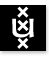Axiomatic Set Theory (Axiomatische Verzamelingentheorie) 2002/2003; 3rd Trimester Institute for Logic, Language & Computation Universiteit van Amsterdam
Instructor: Dr Benedikt Löwe
Vakcode: WI390063
Time: Monday 11-1, Thursday 1-2 12-2
NOTE: Starting with May 22nd, we extended the Thursday Lecture to 12-2.
Place: P.015A
Course language: English
Intended Audience: Mathematics students in their third or fourth year, MoL students

Set Theory is both an area of mathematics (the study of sets as a kind of mathematical object) and an area of mathematical logic (the study of axiom systems of set theory as special axiomatic frameworks). As an area of mathematics, Set Theory has applications in all areas of pure mathematics, most notably set-theoretic topology. (Students planning to specialize in this research area, for example in the Department of Geometry at the Vrije Universiteit will greatly benefit from having a firm understanding of the basics of Set Theory.)

This course will cover the basics of axiomatic set theory presented in a mathematical fashion. Knowledge of logic is not a prerequisite, though familiarity with the axiomatic method is. We shall keep close to the newest edition of Jech's textbook Set Theory:

Thomas Jech, Set Theory, The Third Millenium Edition, revised and expanded, Springer-Verlag 2003.

Topics covered so far:

• Axioms of Set Theory
• Ordinal Numbers
• Cardinal Numbers
• Real Numbers
• The Axiom of Choice and Cardinal Arithmetic

A rough plan for the remaining sessions:

• May 26th: Cardinal Arithmetic; Axiom of Regularity
• May 29th: HOLIDAY
• June 2nd: Filters, Ultrafilters and stationary sets;
• June 5th: Stationary sets
• June 9th: HOLIDAY
• June 12th: Partition Properties and Partition Cardinals; Measurable Cardinals;